NEW!## (Chemistry Paper-II)

Exam Name : UPSC Geo-Scientist and Geologist Exam

Subject : UPSC Geo-Scientist and Geologist Exam Papers 2020 : Geology Paper- II

Year : 2020

## ChemistryPaper - II

• Time Allowed : Three Hours
• Maximum

1. Consider the following statements regarding radial and total angular function of H-atom:
1. The radial function R(r) depends on the quantum number and
2. The total angular function depends only on the direction and is independent of the distance from the nucleus (r)
Which of the statements given  above is/are correct?
(a) 1 only
(b) 2 only
(c) Both 1 and 2
(d) Neither 1 nor 2

2. Which one of the following is the correct electronic configuration of gold (ground state)?
(a) [Xe] 6s2 4f14 5d9
(b) [Xe] 6s1 4f14 5d10
(c) [Xe] 6s2 4f13 5d10
(d) [Xe] 6s2 4f4 5d10

3. Which one of the following is the correct order of increasing acidic nature of oxides?
(a) SiO2 < Al2O3 <SO3 < Cl2O7
(b) SiO2 < SO3 <Al2O3 < Cl2O7
(c) Al2O3 <SiO2 < SO3 < Cl2O7
(d) Cl2O7 < SiO2 < SO3 < Al2O3

4. Which one of the following is the correct outermost electronic configuration of representative elements?
(a) (n-1)d1-10 ns0-2
(b) ns1-2 to ns2 np1-6
(c) (n-2)f1-14 (n-1)d0-1 ns2
(d) ns0.

5. The name of the element given by IUPAC with atomic number 118 is:
(a) Nihonium
(b) Moscovium
(c) Oganesson
(d) Tennessine

6. Which one of the following statements regarding actinoid metals is NOT correct?
(a) The actinoid metals are usually silvery in appearance
(b) Ionization enthalpies of the early actinoids are higher than that of the early lanthanoids
(c) The actinoids are highly reactive metals
(d) The actinoid metals in general show +3 oxidation state

7. Which one of the following is the correct order of ionic radius?
(a) F- > O2– > Na+
(b) Na+ > F- > O2–
(c) H > H > H+
(d) Al3+ > Mg2+ > N3–

8. Which one among the following elements has the highest second ionization potential?
(a) B
(b) Be
(c) Mg
(d) Al

9. Consider the following statements regarding magnetism of two complexes:
1. [CoF6] 3– is a paramagnetic and high spin complex
2. [Co(NH3)6] 3+ is a diamagnetic and low spin complex
Which of the statements given above is/are correct?
(a) 1 only
(b) 2 only
(c) Both 1 and 2
(d) Neither 1 nor 2

10. Consider the following statements in respect of alkali metal halides:
1. The low solubility of LiF in water is due to its high lattice enthalpy
2. The low solubility of CsI in water is due to smaller hydration enthalpy of its two ions
Which of the statements given above is/are correct?
(a) 1 only
(b) 2 only
(c) Both 1 and 2
(d) Neither 1 nor 2

11. When the radius ratio in an ionic crystal lies between 0.732 – 0.999, the coordination number will be:
(a) 3
(b) 4
(c) 6
(d) 8

12. Which one of the following is the correct order of thermal stabilities of metal carbonates?
(a) BaCO3 > CaCO3 > MgCO3 > BeCO3
(b) BaCO3 > MgCO3 > CaCO3 > BeCO3
(c) CaCO3 > BaCO3 > MgCO3 > BeCO3
(d) BaCO3 > BeCO3 > MgCO3 > CaCO3

13. Which one of the following is the correct order of increasing covalent character of halides of Al3+?
(a) AlF3 < AlCl3 < AlBr3 < AlI3
(b) AlI3 < AlBr3 < AlCl3 < AlF3
(c) AlF3 < AlBr3 < AlCl3 < AlI3
(d) AlCl3 < AlF3 < AlBr3 < AlI3

14. The appearance of colour in solid alkali metal halides is generally due to:
(a) Schottky defect
(b) Frenkel defect
(c) Interstitial Position
(d) F-Centres

15. Match List-I with List-II and select the correct answer using the code given below the lists:
List-I(Molecule/ion)     List-II (Shape)
A. H2O             1. Linear
B. BF3             2. Angular
C. I3                3. Trigonal planar
D. SF4             4. See-saw
Code:
A     B     C     D
(a)    4     1     3     2
(b)    2     3     1     4
(c)    2     1     3     4
(d)    4     3     1     2

16. In which of the following molecules/ions, all the bond lengths are NOT equal?
(a) BF3
(b) BF4
(c) XeF4
(d) SF4

17. Which one of the following exhibits highest bond order?
(a) O2
(b) O2
(c) O22–
(d) O2+

18. How many resonating structures are possible for NO3 ion?
(a) 4
(b) 5
(c) 6
(d) 3

19. Consider the following statements for the  complex [Ni(NH3)6]Cl2:
1. It is an outer orbital octahedral complex
2. Hybridization of Ni is sp3d2
3. It is a paramagnetic complex
4. It is a diamagnetic complex
Which of the statements given above are correct?
(a) 1 and 2 only
(b) 2 and 3 only
(c) 1, 2 and 3
(d) 1, 2 and 4

20. Sodium nitroprusside when added to an alkaline solution of sulphide ion gives red coloured solution. The red colouration is due to the formation of:
(a) Na4[Fe(CN)5(NOS)]
(b) Na2[Fe(CN)5(NO)]
(c) Na4[FeCl5(NOS)]
(d) [Fe(H2O)5(NO)]SO4

21. The complex ions [NiCl4]2– and [Ni(CN)4]2– are:
(a) Both square planar
(b) Both diamagnetic
(c) Both paramagnetic
(d) Paramagnetic and diamagnetic respectively

22. Consider the following complexes:
1. [Fe(H2O)6] 2+
2. [Fe(CN)6]3–
3. [Fe(CN)6]4–
4. [Fe(H2O)6]3+
Which one of the following is the correct order of number of unpaired electrons in the above complexes?
(a) 4 > 1 > 2 > 3
(b) 1 > 2 > 3 > 4
(c) 3 > 2 > 1 > 4
(d) 4 > 2 > 1 > 3

23. The denticity of ligands glycinate, iminodiacetic acid and nitrilotriacetic acid are:
(a) 2, 3 and 4 respectively
(b) 3, 3 and 4 respectively
(c) 2, 3 and 3 respectively
(d) 1, 2 and 3 respectively

24. Which one of the following is the IUPAC  name of the complex K3[Fe(CN)6]?
(a) Potassium hexacyanoferrate(II)
(b) Potassium hexacyanoferrate(III)
(c) Trispotassium hexacyanoiron(III)
(d) Potassium hexacyanoiron(III)

25. Which one of the following complexes will NOT show geometrical isomerism?
(a) [Cr(NH3)4Cl2]Cl
(b) [Co(en)2Cl2]Cl
(c) [Co(NH3)5NO2]Cl2
(d) Pt(NH3)2Cl2

26. Which one of the following complexes exhibits optical isomerism?
(a) cis-[PtCl2(NH3)2]
(b) trans-[PtCl2(NH3)2]
(c) trans-[Co(en)2Cl2]+
(d) [Co(EDTA)]

27. Consider the following complex ions:
1. [Co(en)3]3+
2. cis-[Co(en)2Cl2]+
3. trans-[Co(en)2Cl2]+
Which of the complex ion(s) given above show(s) optical activity?
(a) 1 only
(b) 1 and 2
(c) 1 and 3
(d) 2 and 3

28. A solution is obtained by mixing 10 ml  of 0.2 N KOH solution with 30 ml of 0.1 N CH3COOH. The pH of the solution would be: (Given: pKa of CH3COOH = 4.75; log 2 = 0.30)
(a) 5.301
(b) 5.05
(c) 4.699
(d) 4.398

29. What will be the hydrogen ion concentration of a solution obtained by mixing 500 ml of 0.20 M acetic acid and 500 ml of 0.30 M sodium acetate? (Dissociation constant of acetic acid = 1.75 × 10–5 )
(a) 2.75 × 10–5 mol.dm–3
(b) 1.17 × 10–5 mol.dm–3
(c) 3.50 × 10–5 mol.dm–3
(d) 1.75 × 10–3 mol.dm–3

30. Which one of the following represents the  correct order of acid strength?
(a) [Fe(H2O)6]2+ < [Al(H2O)6]3+ < [Fe(H2O)6]3+
(b) [Fe(H2O)6]2+ < [Fe(H2O)6]3+ < [Al(H2O)6]3+
(c) [Fe(H2O)6]3+ < [Fe(H2O)6]2+ < [Al(H2O)6]3+
(d) [Fe(H2O)6]3+ < [Al(H2O)6]3+ < [Fe(H2O)6]2+

31. Consider the following hydroxides:
1. Be(OH)2
2. Sr(OH)2
3. Mg(OH)2
4. Ba(OH)2
The correct order of basicity of the  above hydroxides will be:
(a) Sr(OH)2 < Be(OH)2 < Ba(OH)2 < Mg(OH)2
(b) Be(OH)2 < Mg(OH)2 < Ba(OH)2 < Sr(OH)2
(c) Mg(OH)2 < Ba(OH)2 < Sr(OH)2 < Be(OH)2
(d) Be(OH)2 < Mg(OH)2 < Sr(OH)2 < Ba(OH)2

32. Which one of the following is the correct order of Lewis Acidity in Boron halides?
(a) BF3 < BI3 < BBr3 < BCl3
(b) BI3 < BF3 < BCl3 < BBr3
(c) BF3 < BCl3 < BBr3 < BI3
(d) BBr3 < BI3 < BF3 < BCl3

33. Which one of the following statements regarding acid-base property is NOT correct?
(a) pH + pOH = 14, for all aqueous solutions
(b) The pH of 10–8
(M) HCl solution is 8
(c) The conjugate base of H2PO4 is HPO42–
(d) H2SO4 is a strong acid in aqueous solution

34. What will be the value of pOH of 0.03M aqueous solution of HCl at 25°C? (Given log 0.03 = –1.52)
(a) 12.21
(b) 12.48
(c) 11.48
(d) 10.48

35. What will be the value of mole fraction of the solute in 1.00 molal aqueous solution?
(a) 1.7700
(b) 1.7770
(c) 0.0180
(d) 0.0344

36. Equivalent weight of K2Cr2O7 in acidic medium will be: (Atomic weight of K = 39, Cr = 52 and O = 16)
(a) 294 / 2
(b) 294/ 3
(c) 294/ 6
(d) 294

37. Which one of the following is a primary  standard substance?
(a) KMnO4
(b) K2Cr2O7
(c) Na2S2O3
(d) NaOH

38. The equivalence point of titration of oxalate solution (acidic medium) with KMnO4 solution will be:
(a) Pink
(b) Colourless
(c) Orange
(d) Light green

39. In which of the following titrations, an external indicator is NOT necessary?
(a) Titration of oxalate solution with KMnO4 solution
(b) Titration of HCl with NaOH solution
(c) Titration of Hard water with EDTA
(d) Titration of Na2CO3 with HCl solution

40. Which one among the following is a metal ion type indicator?
(a) Methylene blue
(b) Diphenylamine sulphonic acid
(c) Eriochrome black T
(d) Cresol red

41. At what temperature does the total kinetic energy of 0.3 mole of Helium equals the total kinetic energy of 0.4 mole of Argon at 400 K?
(a) 533.3 K
(b) 433.3 K
(c) 346.3 K
(d) 373.0 K

42. The ratio of Boyle’s temperature (TB) and critical temperature (TC) of a gas obeying van der Waals equation is given by:
(a) TB : TC = 27: 8
(b) TB : TC = 4: 27
(c) TB : TC = 3: 2
(d) TB : TC = 8: 27

43. Weight of O2 necessary to fill up a cylinder of 0.082 litre capacity at 0˚C and 96 atm pressure would be: (Compressibility factor = 0.96 and gas constant (R) = 0.082 dm3 atm K-1 mol-1 )
(a) 11.72 g
(b) 1.172 g
(c) 117.2 g
(d) 10.72 g

44. Which one of the following gas samples will have the highest average molecular speed? (Atomic weight of O = 16, Ne = 20, C = 12 and He = 4)
(a) 1.0 mole of O2 at 560 K
(b) 0.50 mole of Ne at 500 K
(c) 0.20 mole of CO2 at 440 K
(d) 2.0 mole of He at 140 K46. The amount of energy (per mole of a monoatomic gas) that will increase by increasing the temperature by one degree at constant volume is:
(a) Cp – Cv
(b) R/2
(c) 3/2 R
(d) Cv + R

47. Which one among the following gases has the largest mean free path under the same external conditions?
(a) H2
(b) Cl2
(c) N2
(d) O2

48. 50 mL of H2 gas diffuses through a small hole from a vessel in 20 minutes. Time taken by 40 mL of O2 gas to diffuse under similar condition will be:
(a) 12 minutes
(b) 64 minutes
(c) 8 minutes
(d) 32 minutes

49. What will be the slope of the plot of log P vs log V for a reversible adiabatic expansion of an ideal gas? [γ = Cp/Cv]
(a) γ
(b) – γ
(c) zero
(d) –1

50. The latent heat of vaporization of Br2 at 59˚C and at 1 atm pressure is 29.2 kJ mol-1 . The value of ∆U would be: (R = 8.314 × 10-3 kJ mol-1 K-1 )
(a) 26.44 kJ mol-1
(b) 2.644 kJ mol-1
(c) 264.4 kJ mol-1
(d) 31.96 kJ mol-1

51. The ratio of specific heat of gases at constant pressure (Cp) and constant volume (Cv) of N2 molecule is:
(a) 1.667
(b) 1.400
(c) 1.330
(d) 1.520

52. The total heat content of a system at constant pressure is known as:
(a) Enthalpy
(b) Entropy
(c) Internal energy
(d) Activation energy

53. In the Joule-Thomson expansion of real gas at inversion temperature, the Joule-Thomson coefficient:
(a) will be negative if the gas cools post expansion
(b) will be positive if the gas gets heated post expansion
(c) will be zero if [∂(PV)/ ∂P]T is positive
(d) is zero

54. For N2(g) + 3H2(g) ⇋ 2 NH3(g), the expression for ∆H is:
(a) ∆U + 2 RT
(b) ∆U + RT
(c) ∆U – 2 RT
(d) ∆U – RT

55. The entropy of vaporization of a liquid is 100  J mol-1K-1 and it boils at 300K. What would be the value of ∆Hvap
(a) 3.0 kJ mol-1
(b) 30.0 kJ mol-1
(c) 300 kJ mol-1
(d) 3000 kJ mol-1

56. The maximum efficiency of a Carnot engine operating between 100˚C to 200˚C will be:
(a) 21.85 %
(b) 21.14 %
(c) 22.14 %
(d) 20.14%

57. Which one of the following is the correct thermodynamic equation of state?
(a) dG = (∂G/∂P)T dP + (∂G/∂T)P dT
(b) (∂U/∂V)T = P + T (∂P/∂T)V
(c) dU = (∂U/∂V)S dV + (∂U/∂S)dS
(d) (∂H/∂P)T = V–T (∂V/∂T)P, n

58. Which one of the following changes will necessarily lead to a non-spontaneous change?
(a) Positive ∆H and positive ∆S
(b) Negative ∆H and negative ∆S
(c) Positive ∆H and negative ∆S
(d) Negative ∆H and positive ∆S

59. Equal volumes of what concentrations of AgNO3 and HCl should be mixed to form a AgCl precipitate? [Ksp for AgCl = 10-10]
(a) 10-4 M AgNO3 and 10-7 M HCl
(b) 10-5 M AgNO3 and 10-6 M HCl
(c) 10-6 M AgNO3 and 10-6 M HCl
(d) 10-4 M AgNO3 and 10-5 M HCl

60. The solubility product of Ag2CrO4 is 32 × 10–12.  What is the concentration of CrO42– ion in that solution?
(a) 2 × 10-4 M
(b) 16 × 10-4 M
(c) 8 × 10-4 M
(d) 8 × 10-8 M

61. What will be the degree of dissociation of 0.1 (M) Ba(NO3)2 solution, if van’t Hoff factor of the solution is 2.74?
(a) 91.3 %
(b) 87 %
(c) 100%
(d) 74%

62. Which one of the following colligative properties can provide the most precise molar mass of peptides?
(a) Elevation of boiling point
(b) Depression of freezing point
(c) Relative lowering of vapour pressure
(d) Osmotic pressure

63. At 298 K, the total vapour pressure in Torr for a   mixture of volatile components A and B is given by P = 120–75 XB ( XB is the mole fraction of B). What is the vapour pressure of pure A and B (in Torr) respectively?
(a) 120 and 75
(b) 120 and 195
(c) 120 and 45
(d) 75 and 4565. A 1% aqueous solution (mass by volume) of a certain substance is isotonic with a 3% solution of  glucose (Molar mass = 180 g mol-1 ) at a given temperature. The molar mass of the substance (in kg mol-1 ) will be:
(a) 0.60
(b) 180
(c) 0.18
(d) 0.06

66. Which among the following is the correct order for osmotic pressure of equimolar solutions of glucose, sodium chloride and barium chloride?
(a) C6H12O6 > BaCl2 > NaCl
(b) C6H12O6 > NaCl > BaCl2
(c) BaCl2 > NaCl > C6H12O6
(d) NaCl > C6H12O6 > BaCl2

67. 0.05 molal aqueous solution of K4[Fe(CN)6] freezes at –0.26˚C. Its degree of dissociation will be: (kf =1.86˚C Kg mol-1 )
(a) 48.2 %
(b) 54.9 %
(c) 44.9 %
(d) 51.6 %

68. What will be the value of freezing point depression constant of cyclobutane having freezing point of 280 K and molar enthalpy of fusion of 2.68 kJ mol-1 ? (Molar mass of cyclobutane = 56.108 g mol-1 and R= 8.314 JK-1mol-1 )
(a) 14.63 K kg mol-1
(b) 16.14 K kg mol-1
(c) 13.64 K kg mol-1
(d) 12.64 K kg mol-1

69. Which one of the following statements is correct about the Henry’s law?
(a) It is related to effect of pressure only on solubility of a gas in a liquid
(b) It is related to effect of temperature only on solubility of a gas in a liquid
(c) It is related to effect of pressure as well as temperature on solubility of a gas in a liquid
(d) It is related to effect of pressure as well as temperature on solubility of a gas in a solid

70. What will be the ratio of mole fractions of O2 and N2  dissolved in water at 25˚C? (Given that Henry’s law constant (in torr) for O2 and N2 are 3.3 × 107 and 6.5 × 107 respectively at 25˚C; mole fractions of O2 and N2 in air are taken as 0.2 and 0.8 respectively)
(a) ~ 0.51
(b) ~0.66
(c) ~0.25
(d) ~0.49

71. The cell constant with 0.1 N KCl solution is found to be 0.98 cm-1 and the resistance offered by 0.01 N HCl is 89 Ω. The specific conductance of HCl will be:
(a) 1.101 × 102 Ω -1 cm-1
(b) 1.101 × 10-2 Ω -1 cm-1
(c) 2.202 × 10-3 Ω -1 cm-1
(d) 2.202 × 103 Ω -1 cm-1

72. What will be the correct order of mobility of the following alkali metal ions in aqueous solution?
(a) Li+ > Na+ > K+ > Rb+
(b) Na+ > K+ > Rb+ > Li+
(c) K+ > Rb+ > Na+ > Li+
(d) Rb+ > K+ > Na+  > Li+

73. During electrolysis of silver nitrate solution, loss in the anode compartment was 0.589 g. In a Voltameter connected in series, 1.259 g of silver was deposited. What would be the transport number of two ions?
(a) t+ = 0.468 and t = 0.532
(b) t+ = 0.532 and t = 0.468
(c) t+ = 0.670 and t= 0.330
(d) t+ = 0.330 and t = 0.670

74. The molar conductivities of KOAc and HCl at infinite dilution in water at 25˚C are 90.0 and 426.2 S cm2 mol-1 , respectively. To calculate the molar conductivity of HOAc at infinite dilution, the additional molar conductivity value of which one of the following electrolytes will be required?
(a) NaCl
(b) KCl
(c) KNO3
(d) NaOH

75. What will be the effect of dilution on specific and equivalent conductance of an aqueous solution of a strong electrolyte?
(a) The specific conductance decreases and equivalent conductance increases
(b) The specific conductance increases and equivalent conductance decreases
(c) Both will increase
(d) Both will decrease77. The specific conductance of 0.01 M solution of acetic acid was found to be 0.015 S m-1 at 25˚C. Molar conductance of acetic acid at infinite dilution is 400 × 10-4 S m2 mol-1 . The degree of dissociation of acetic acid will be:
(a) 0.375
(b) 0.0375
(c) 0.00375
(d) 0.357

78. In a moving boundary experiment with 0.01 mole LiCl,  the boundary in a tube with cross sectional area of 0.125 cm2 moves through 8.1 cm in 1500 sec for a current of 1.8 × 10-3 ampere.
What will be the value of transport number of Cl ? (Given that F = 96,500 Coulomb and temperature is 300K)

(a) 0.639
(b) 0.361
(c) 0.510
(d) 0.369

79. When HCl is titrated against NaOH, the conductance at the end point is:
(a) Zero
(b) Minimum
(c) Maximum
(d) Infinite

80. A cell is bathed in a solution that has a greater osmolarity than that of cytosol. The solution can be described as:
(a) Hypertonic
(b) Hypotonic
(c) Isotonic
(d) Hydrotonic83. The o/p-directing effect of methyl group in electrophilic substitution reaction of toluene is due to:
(a) Inductive effect
(b) Hyperconjugation effect
(c) Resonance effect
(d) Electromeric effect87. Classify the following species as electrophiles ( E) and nucleophiles (N) in organic reactions: SO3, Cl+ , NO2+ , CH3NH2, H3O+ , BH3, CN¯
(a) E =SO3, Cl+ , NO2 + , H3O + and N= BH3, CH3NH2, CN¯
(b) E =Cl+ , NO2+ , H3O + and N = SO3, BH3, CH3NH2, CN¯
(c) E =SO3, BH3, Cl+ , NO2 + , H3O  + and N = CH3NH2, CN¯
(d) E =CH3NH2, NO2 + , H3O+ , Cl+ and N = SO3, BH3, CN¯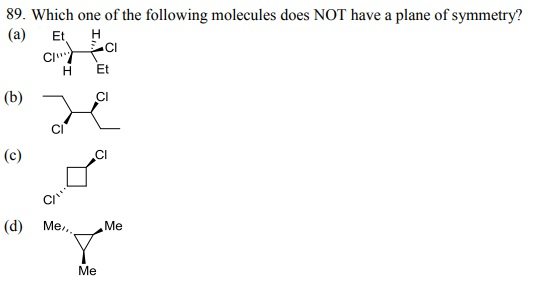90. Which one of the following amino acids is achiral?
(a) Alanine
(b) Glycine
(c) Proline
(d) Valine96. The total number of stereoisomers possible for the given compound is:
ClCH2CH=CHCH=CHCH2CH3
(a) 4
(b) 6
(c) 8
(d) 10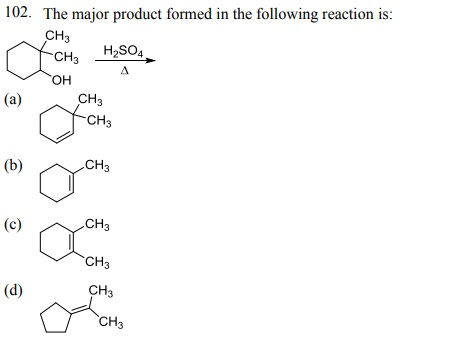104. Which one of the following bromides CANNOT form an alkene by E2 process?
(a) 1-Bromo-2,2-dimethylpropane
(b) 2-Bromo-2-methylbutane
(c) 2-Bromo-3-methylbutane
(d) 1-Bromo-3-methylbutane108. The major product formed  in the reaction of 3-phenylpropene with HBr in the presence of peroxide is:
(a) 2-Bromo-1-phenylpropane
(b) 1,2-Dibromo-3-phenylpropane
(c) 1-Bromo-3-phenylpropane
(d) 3-(p-Bromophenyl) propene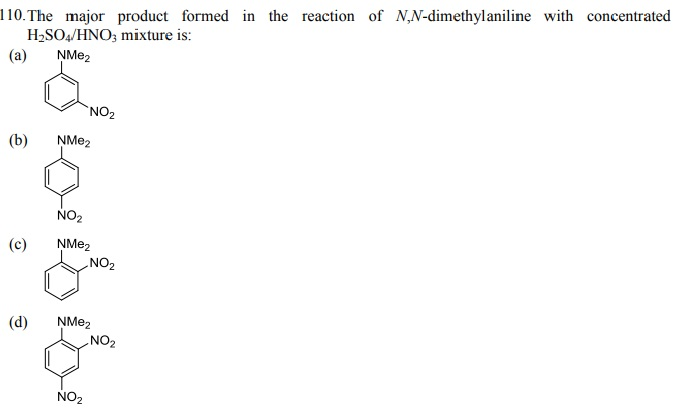114. The product formed by the reaction of  toluene with chlorine in the presence of sunlight is:
(a) o-chlorotoluene
(b) 2,4-dichlorotoluene
(c) p-chlorotoluene
(d) benzylchloride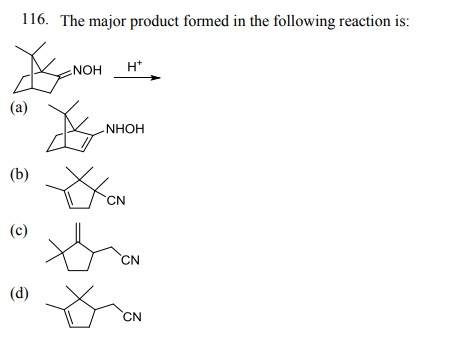## << Go Back To Main Page

Courtesy: UPSC

AttachmentSize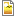upsc-combined-geo-scientist-and-geologist-exam-chemistry-paper-II-2020-img-1.jpg29.65 KBupsc-combined-geo-scientist-and-geologist-exam-chemistry-paper-II-2020-img-2.jpg30.93 KBupsc-combined-geo-scientist-and-geologist-exam-chemistry-paper-II-2020-img-3.jpg44.57 KBupsc-combined-geo-scientist-and-geologist-exam-chemistry-paper-II-2020-img-4.jpg23.35 KBupsc-combined-geo-scientist-and-geologist-exam-chemistry-paper-II-2020-img-5.jpg22.39 KBupsc-combined-geo-scientist-and-geologist-exam-chemistry-paper-II-2020-img-6.jpg24.78 KBupsc-combined-geo-scientist-and-geologist-exam-chemistry-paper-II-2020-img-7.jpg17.58 KBupsc-combined-geo-scientist-and-geologist-exam-chemistry-paper-II-2020-img-8.jpg19.37 KBupsc-combined-geo-scientist-and-geologist-exam-chemistry-paper-II-2020-img-9.jpg19.72 KBupsc-combined-geo-scientist-and-geologist-exam-chemistry-paper-II-2020-img-10.jpg29.68 KBupsc-combined-geo-scientist-and-geologist-exam-chemistry-paper-II-2020-img-11.jpg18.41 KBupsc-combined-geo-scientist-and-geologist-exam-chemistry-paper-II-2020-img-12.jpg27.03 KBupsc-combined-geo-scientist-and-geologist-exam-chemistry-paper-II-2020-img-13.jpg22.23 KBupsc-combined-geo-scientist-and-geologist-exam-chemistry-paper-II-2020-img-14.jpg31.72 KBupsc-combined-geo-scientist-and-geologist-exam-chemistry-paper-II-2020-img-15.jpg34.59 KBupsc-combined-geo-scientist-and-geologist-exam-chemistry-paper-II-2020-img-16.jpg22.61 KBupsc-combined-geo-scientist-and-geologist-exam-chemistry-paper-II-2020-img-17.jpg21.85 KBupsc-combined-geo-scientist-and-geologist-exam-chemistry-paper-II-2020-img-18.jpg23.21 KBupsc-combined-geo-scientist-and-geologist-exam-chemistry-paper-II-2020-img-20.jpg18.17 KBupsc-combined-geo-scientist-and-geologist-exam-chemistry-paper-II-2020-img-21.jpg18.9 KBupsc-combined-geo-scientist-and-geologist-exam-chemistry-paper-II-2020-img-22.jpg34.31 KBupsc-combined-geo-scientist-and-geologist-exam-chemistry-paper-II-2020-img-23.jpg19.39 KBupsc-combined-geo-scientist-and-geologist-exam-chemistry-paper-II-2020-img-24.jpg28.43 KBupsc-combined-geo-scientist-and-geologist-exam-chemistry-paper-II-2020-img-25.jpg21.13 KBupsc-combined-geo-scientist-and-geologist-exam-chemistry-paper-II-2020-img-26.jpg23.36 KBupsc-combined-geo-scientist-and-geologist-exam-chemistry-paper-II-2020-img-27.jpg41.38 KBupsc-combined-geo-scientist-and-geologist-exam-chemistry-paper-II-2020-img-28.jpg24.23 KBupsc-combined-geo-scientist-and-geologist-exam-chemistry-paper-II-2020-img-29.jpg20.91 KBupsc-combined-geo-scientist-and-geologist-exam-chemistry-paper-II-2020-img-30.jpg21.01 KBupsc-combined-geo-scientist-and-geologist-exam-chemistry-paper-II-2020-img-31.jpg21.38 KBupsc-combined-geo-scientist-and-geologist-exam-chemistry-paper-II-2020-img-32.jpg31.26 KBupsc-combined-geo-scientist-and-geologist-exam-chemistry-paper-II-2020-img-33.jpg24.19 KBupsc-combined-geo-scientist-and-geologist-exam-chemistry-paper-II-2020.pdf720.39 KBupsc-combined-geo-scientist-and-geologist-exam-chemistry-paper-II-2020-img-34.jpg17.82 KBupsc-combined-geo-scientist-and-geologist-exam-chemistry-paper-II-2020-img-35.jpg31.66 KBupsc-combined-geo-scientist-and-geologist-exam-chemistry-paper-II-2020-img-36.jpg22.22 KBupsc-combined-geo-scientist-and-geologist-exam-chemistry-paper-II-2020-img-38.jpg26.04 KB